# NCERT Solutions For Class 10 Maths Chapter 5 Exercise 5.3 – Arithmetic Progressions

Download NCERT Solutions For Class 10 Maths Chapter 5 Exercise 5.3 – Arithmetic Progressions. This Exercise contains 20 questions, for which detailed answers have been provided in this note. In case you are looking at studying the remaining Exercise for Class 10 for Maths NCERT solutions for other Chapters, you can click the link at the end of this Note.

### NCERT Solutions For Class 10 Maths Chapter 5 Exercise 5.3 – Arithmetic Progressions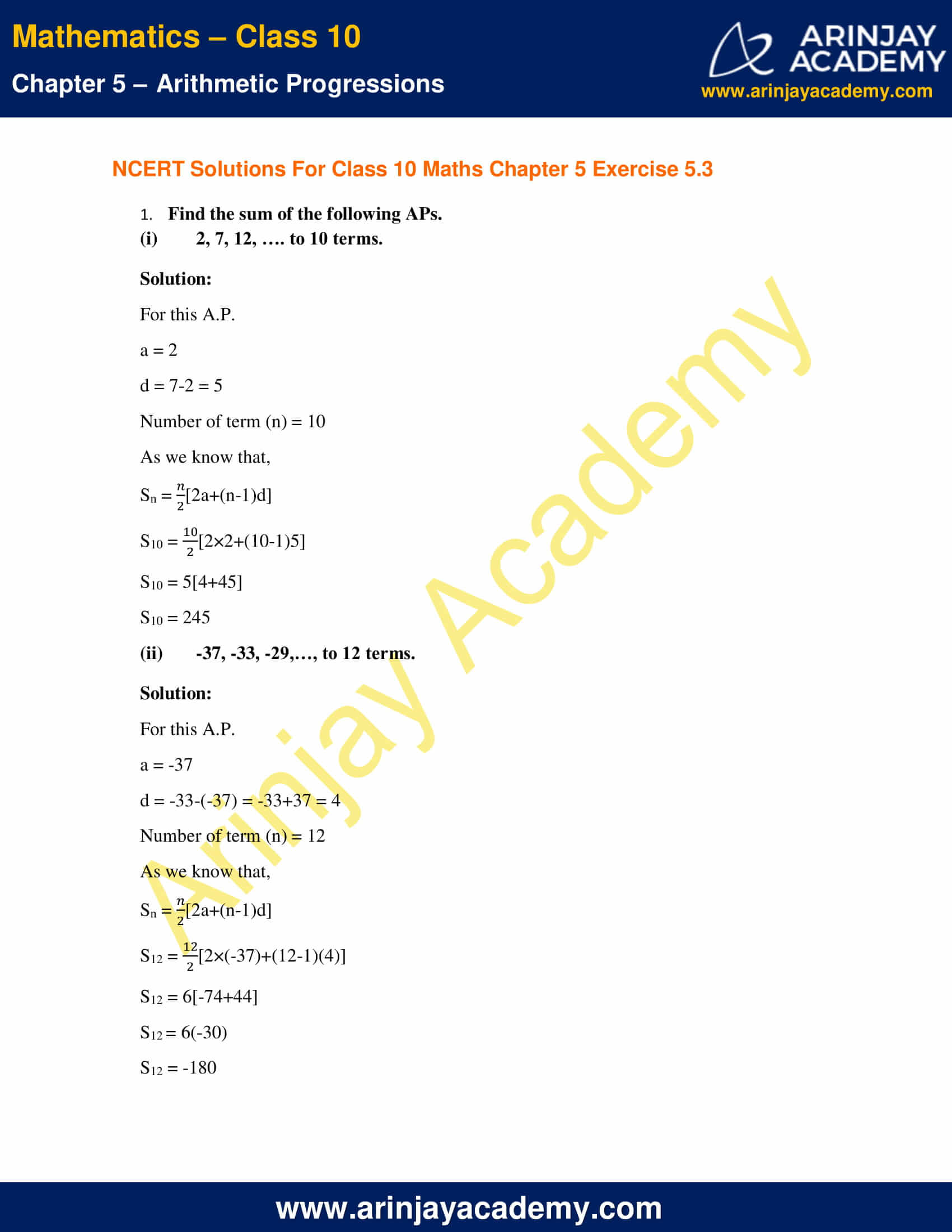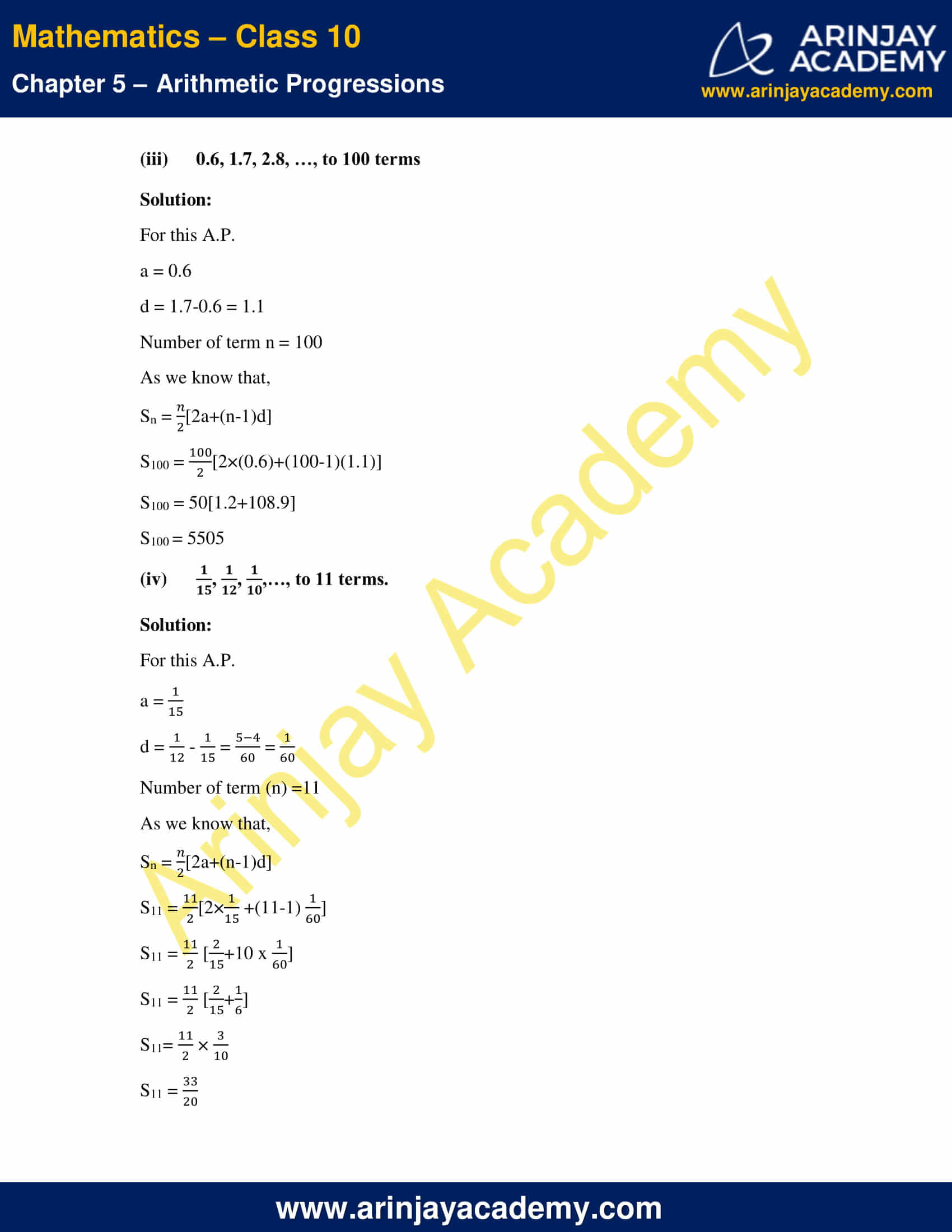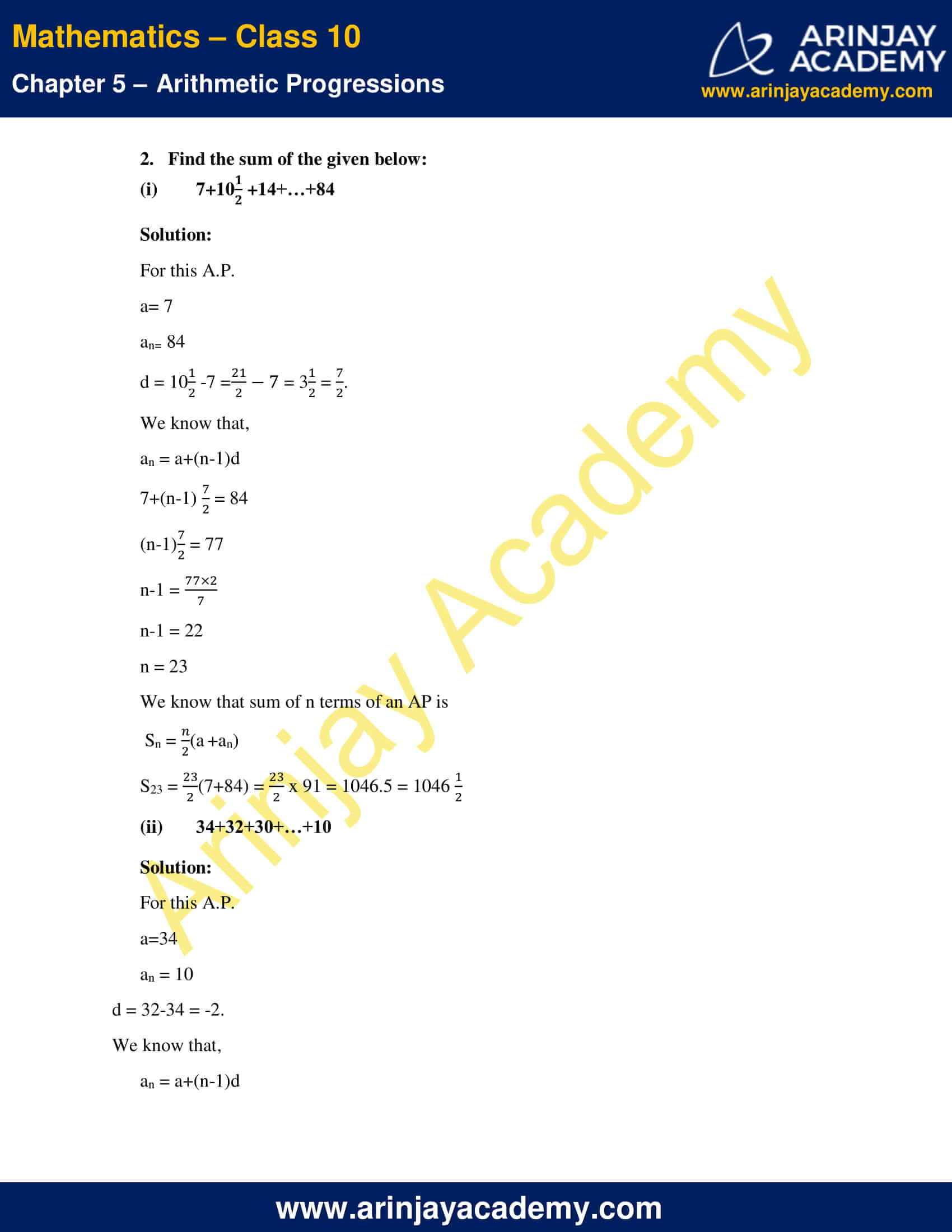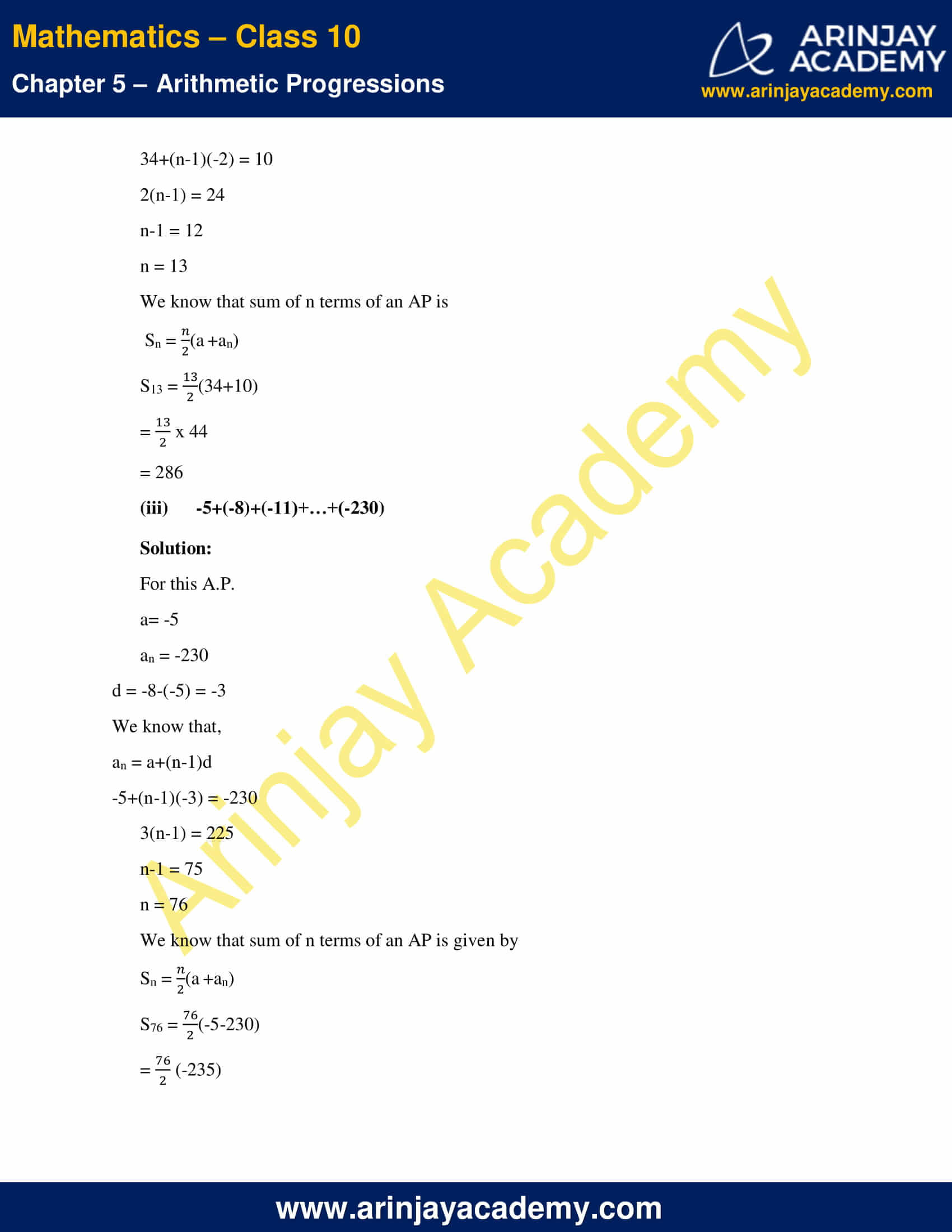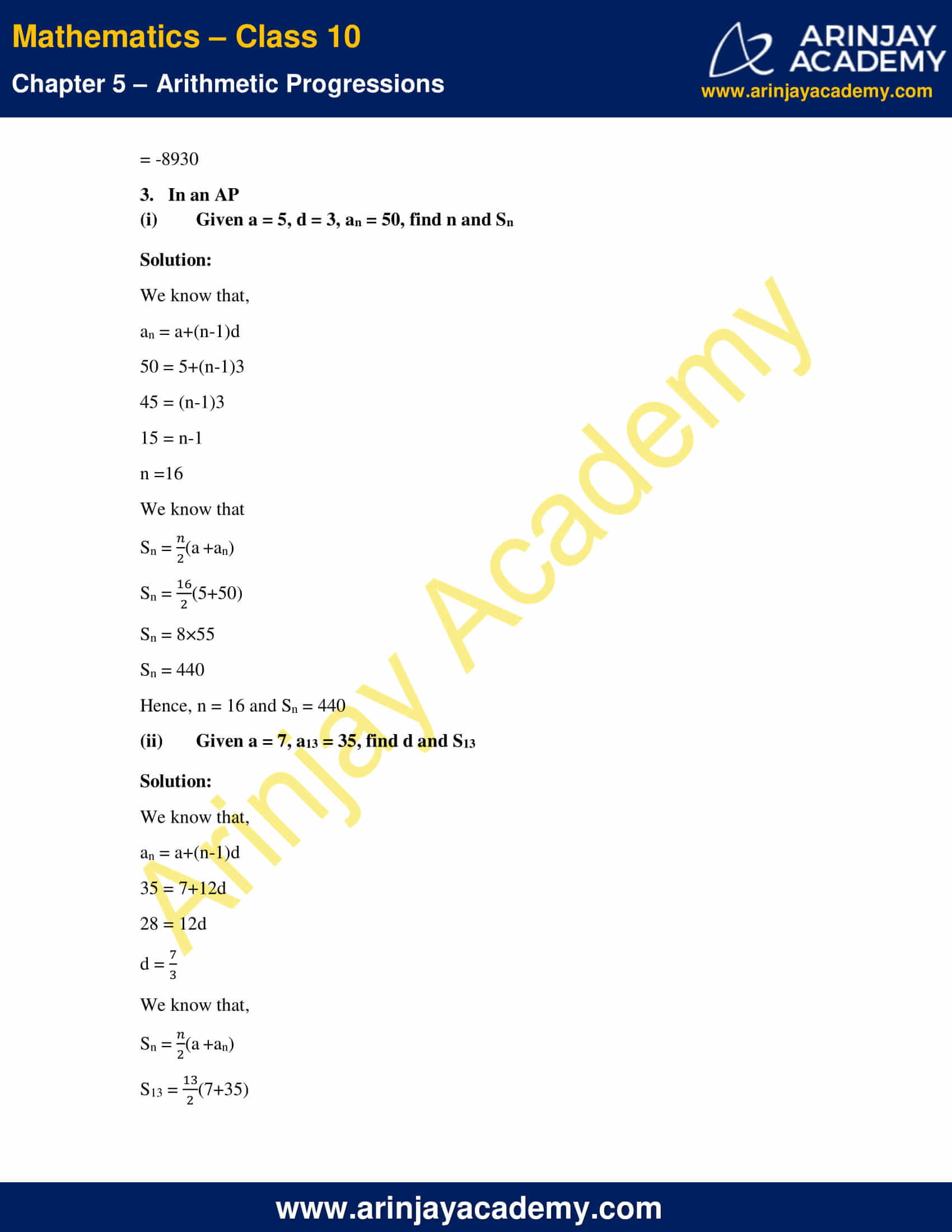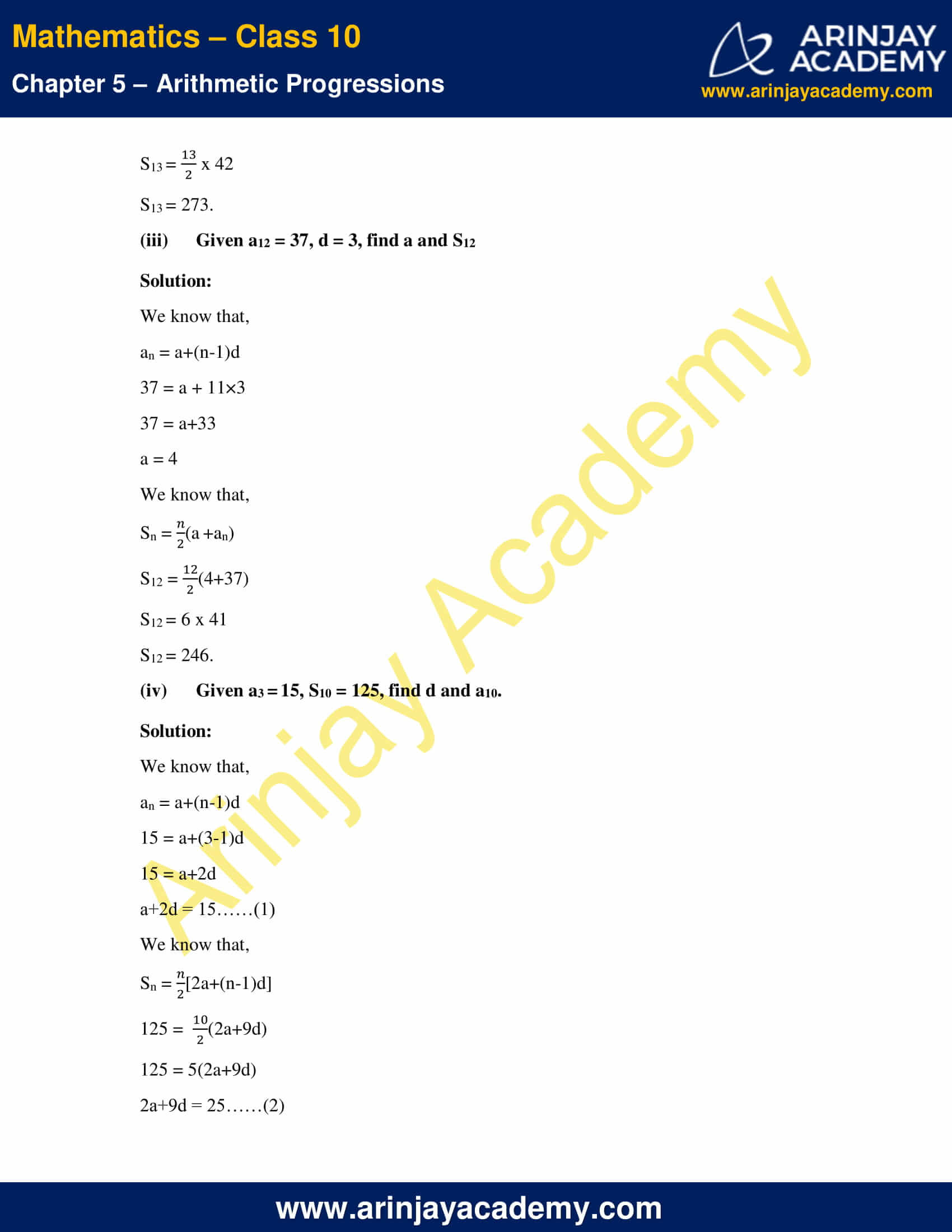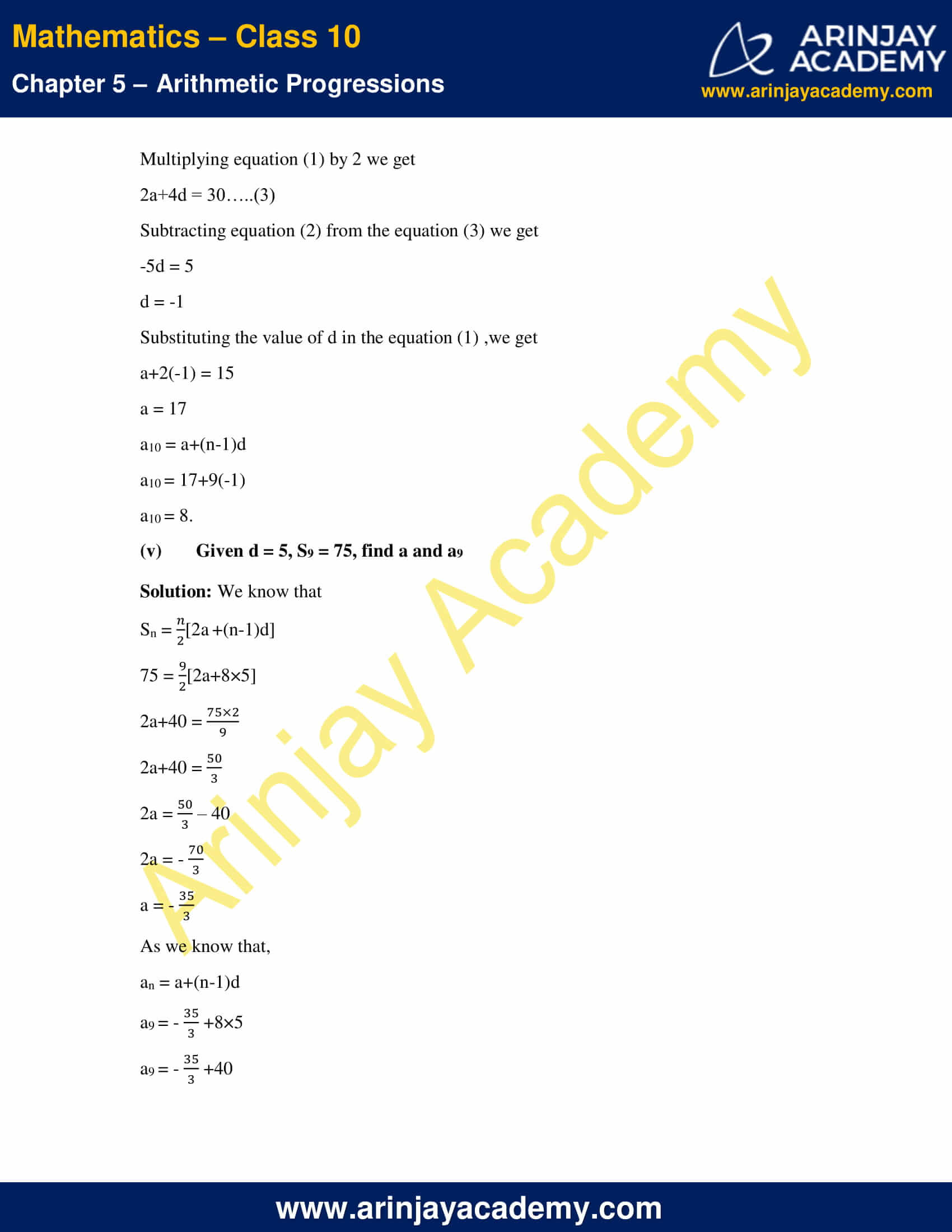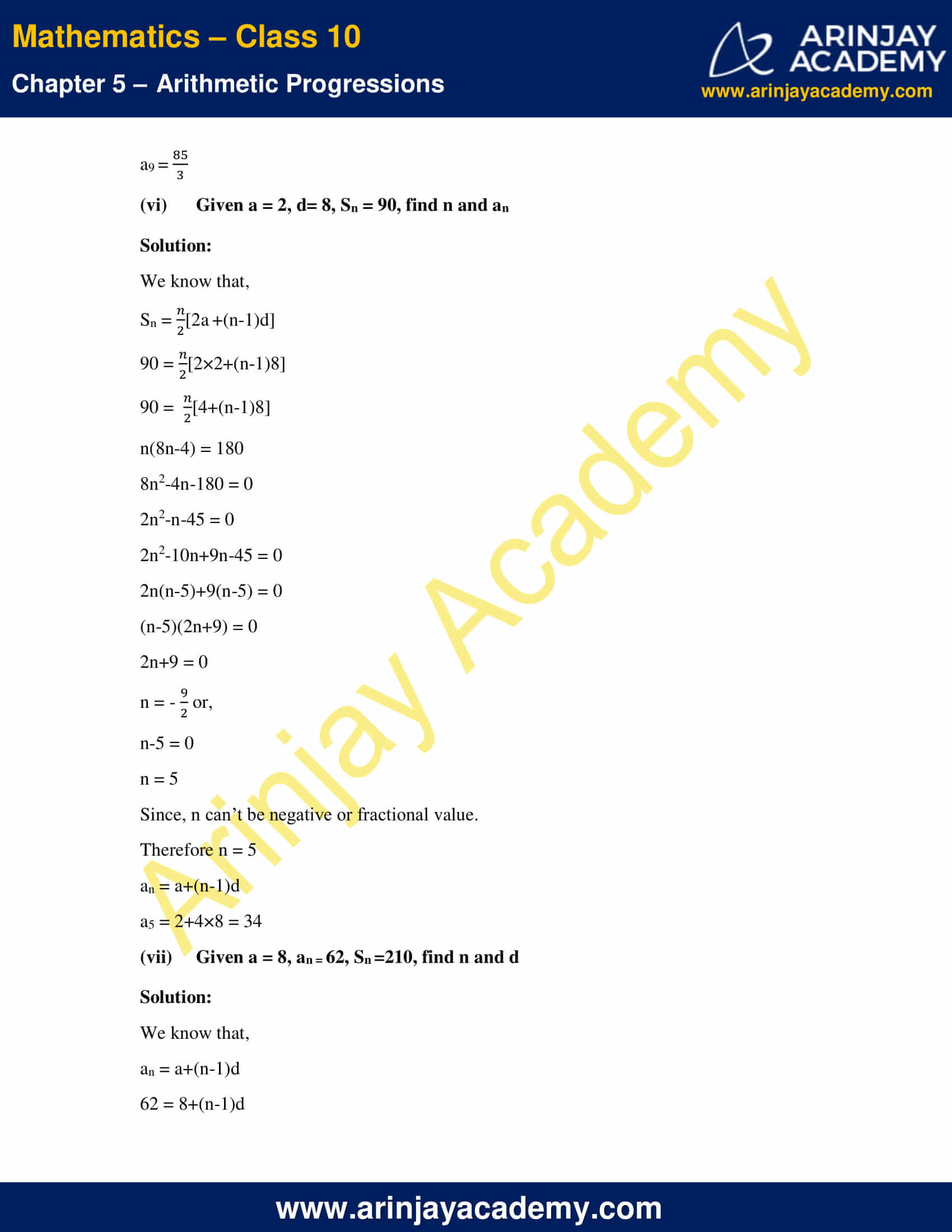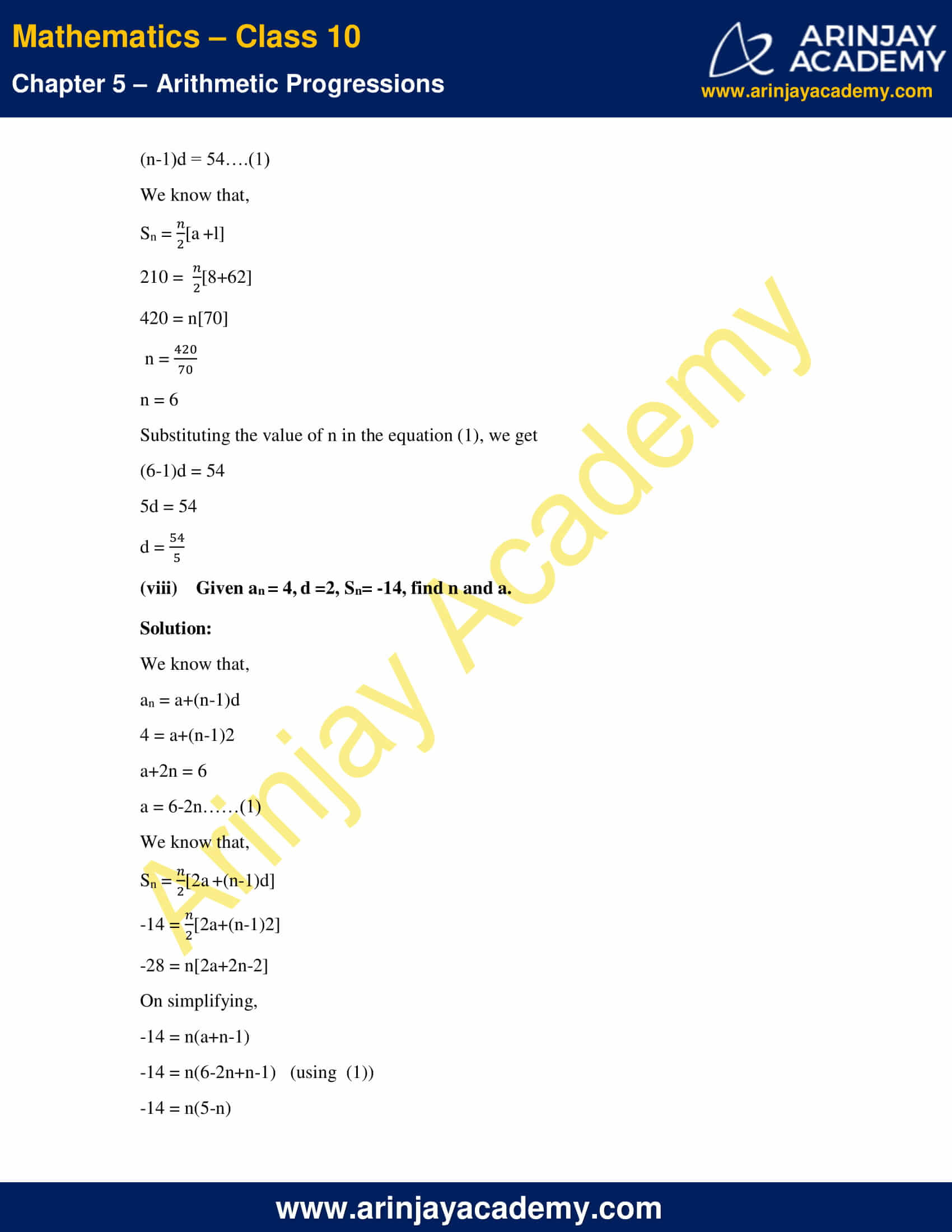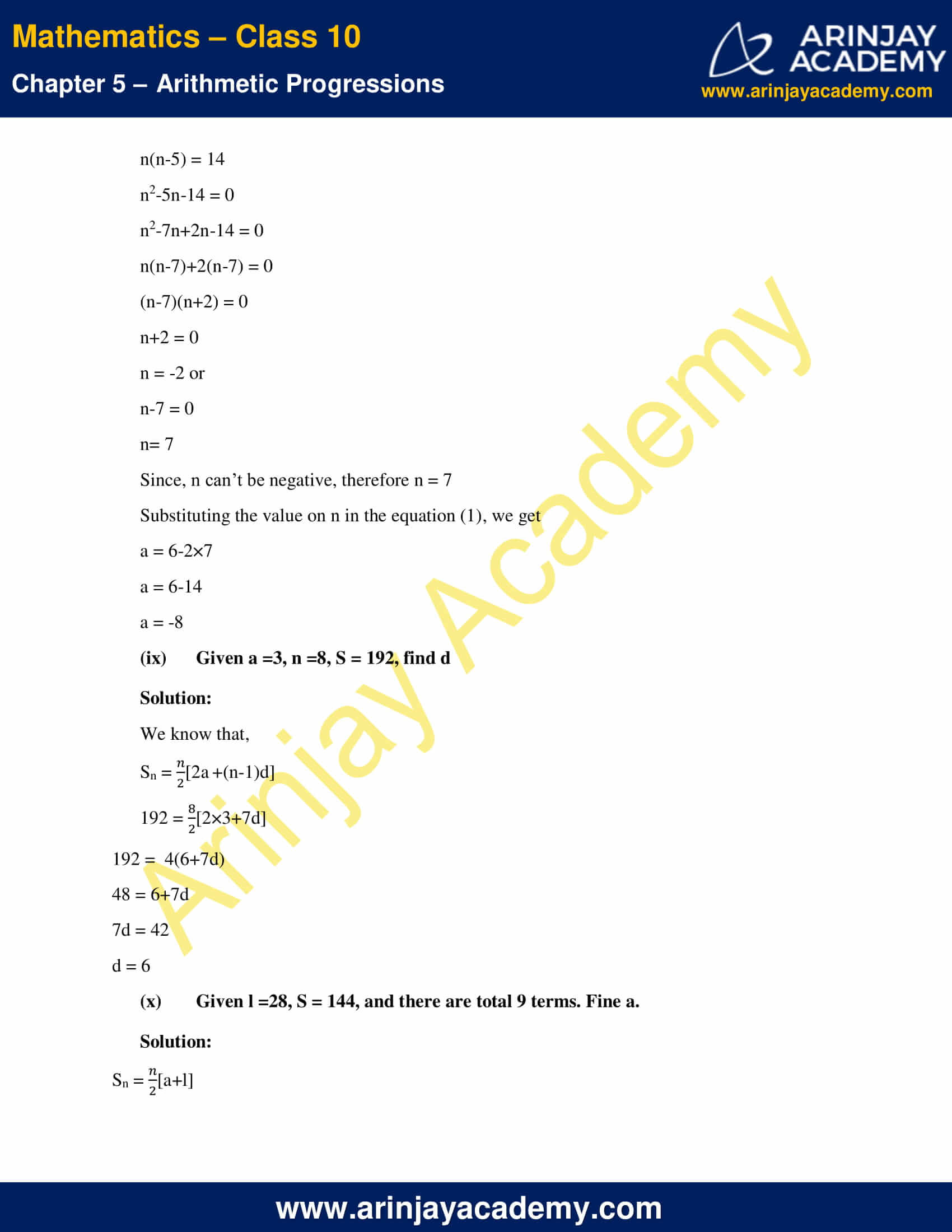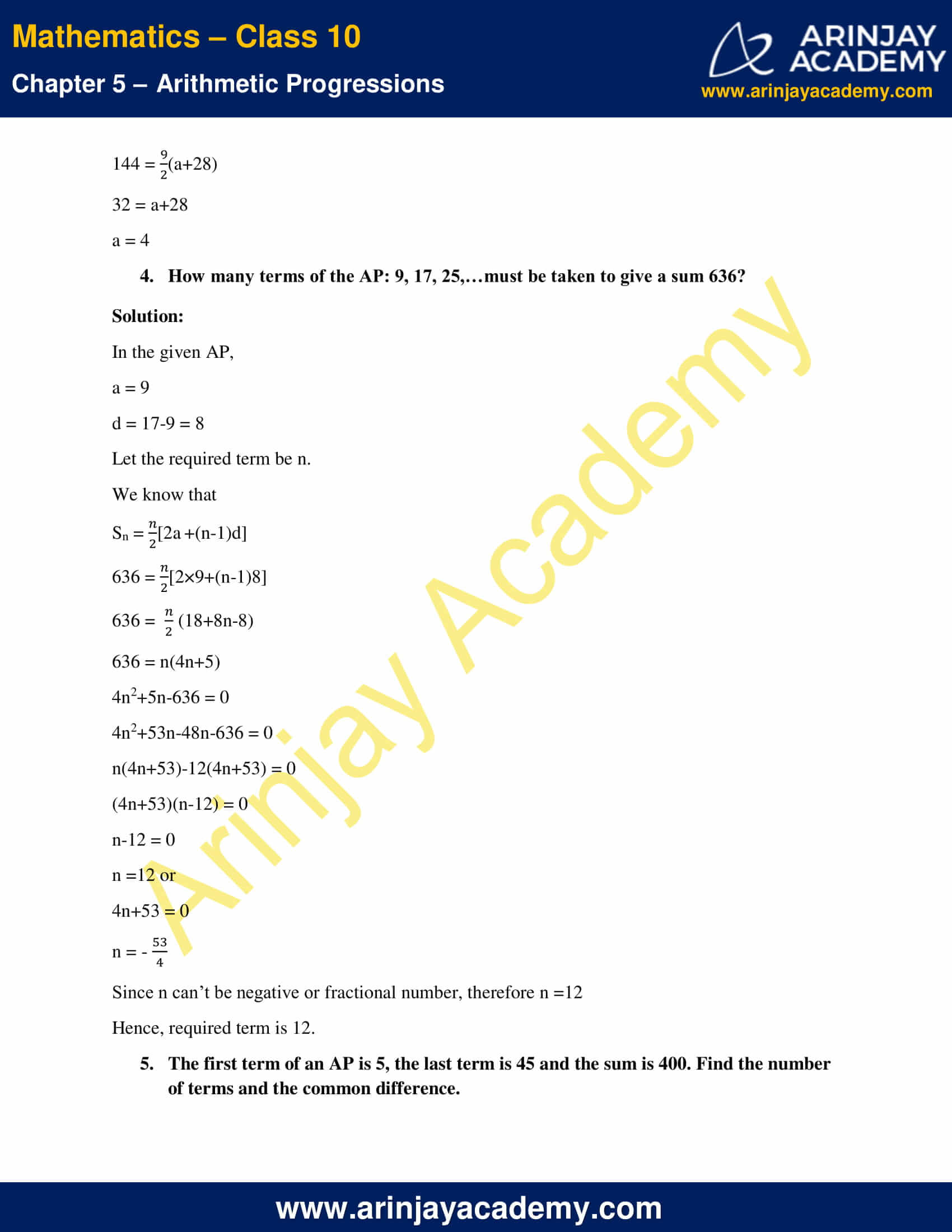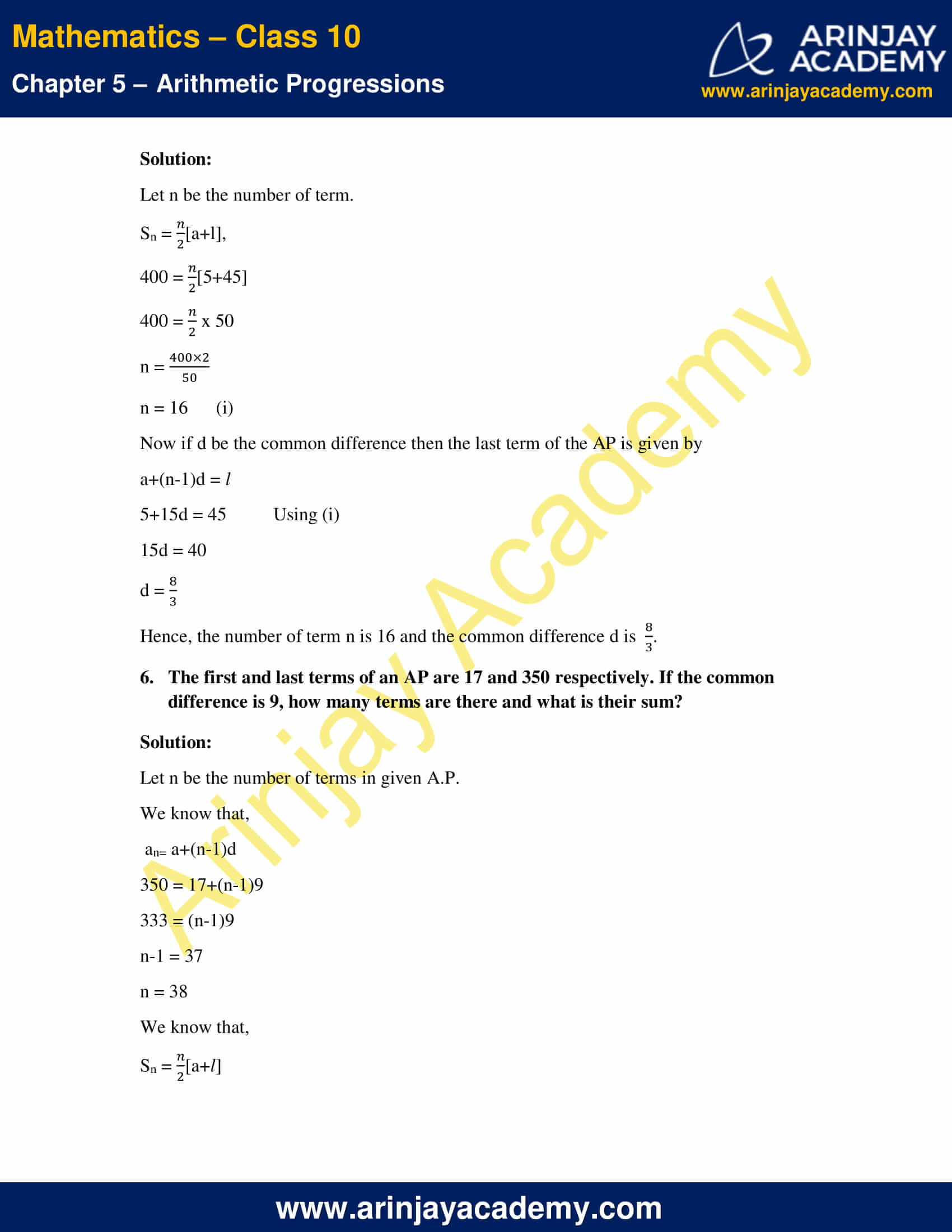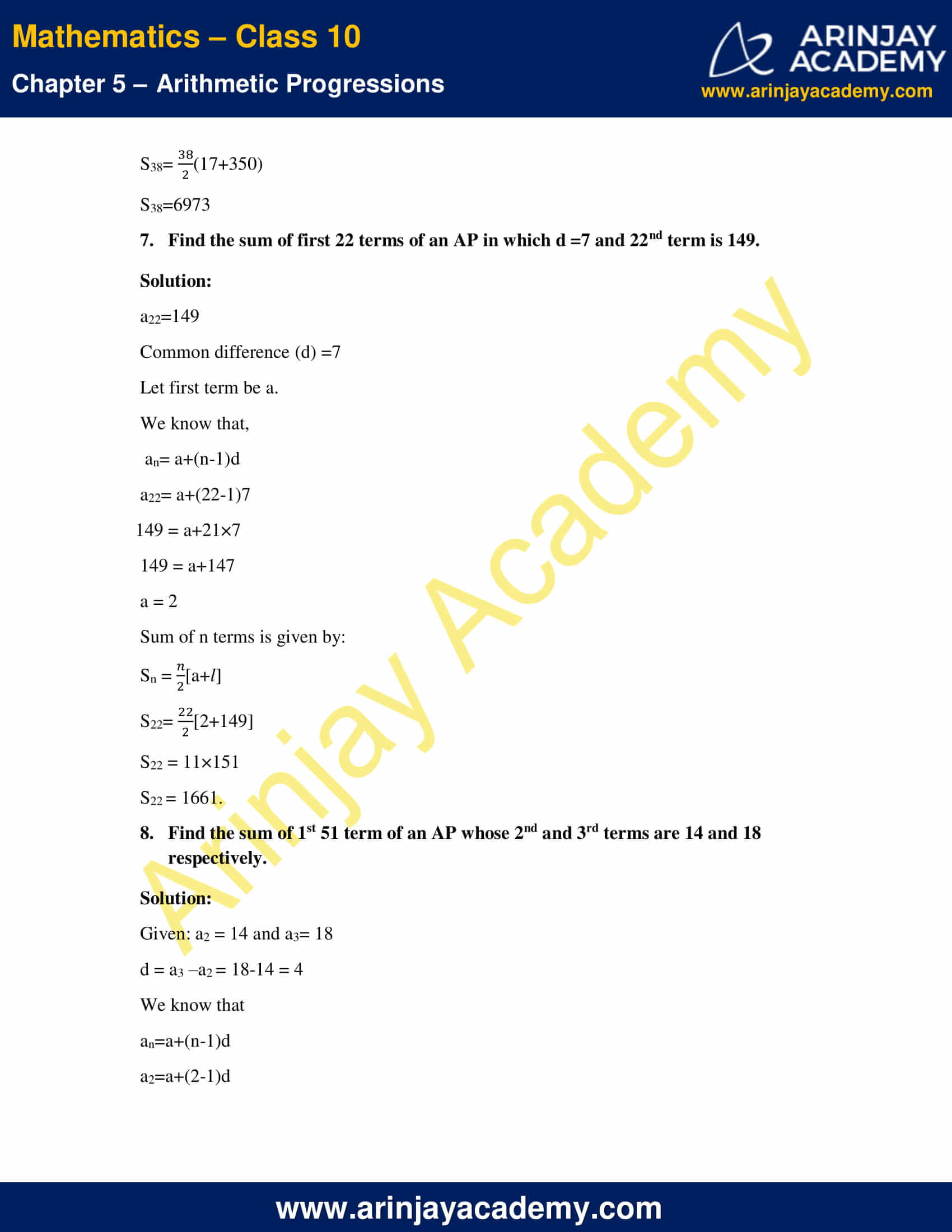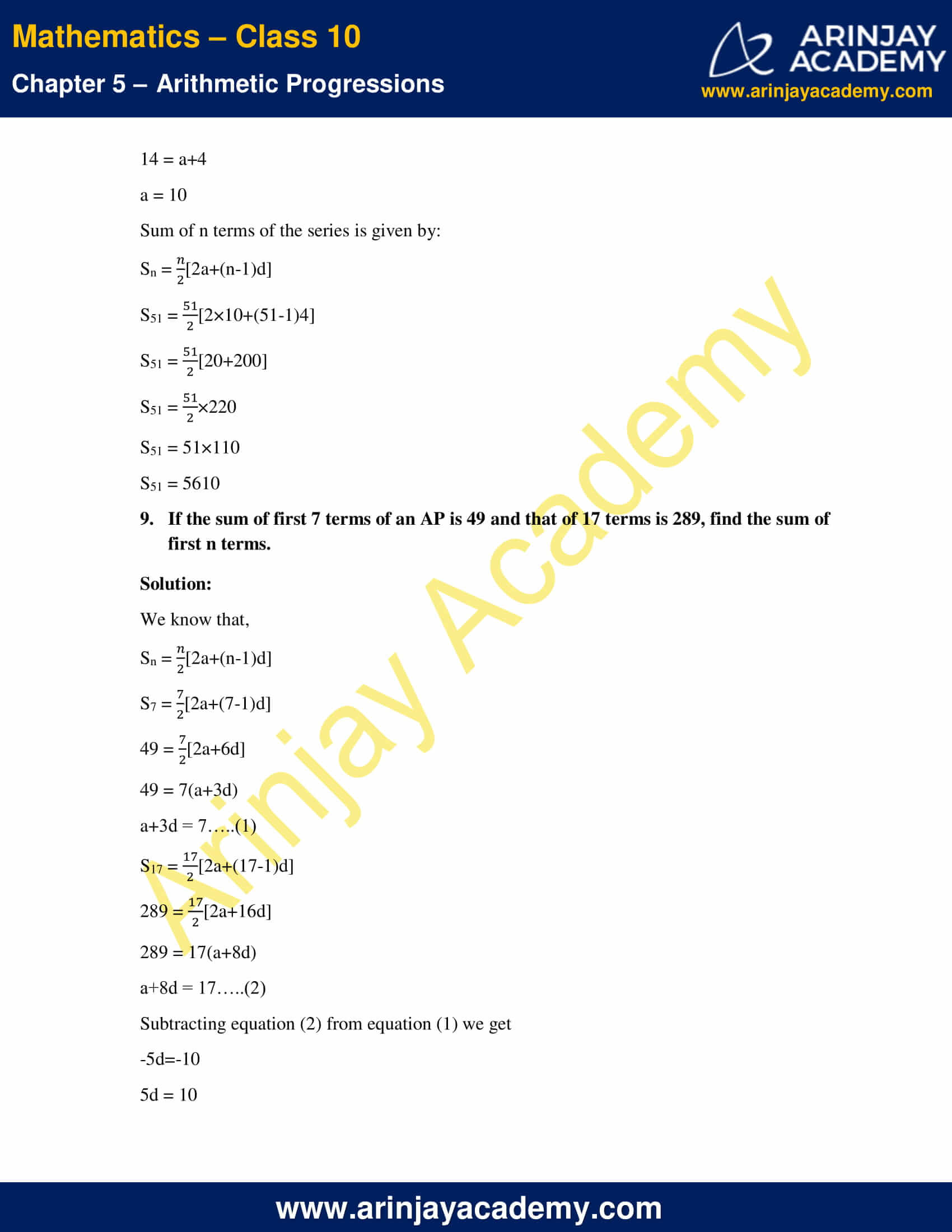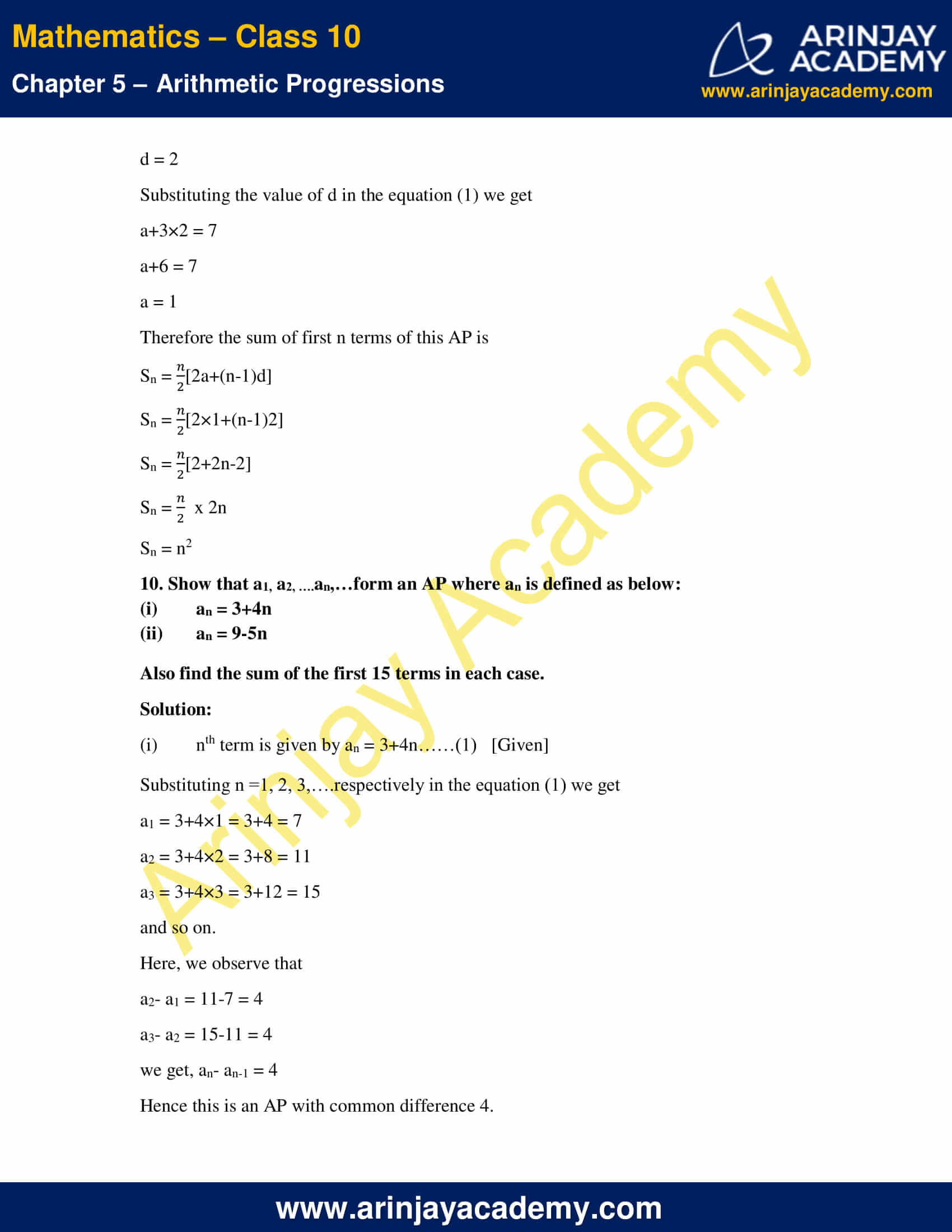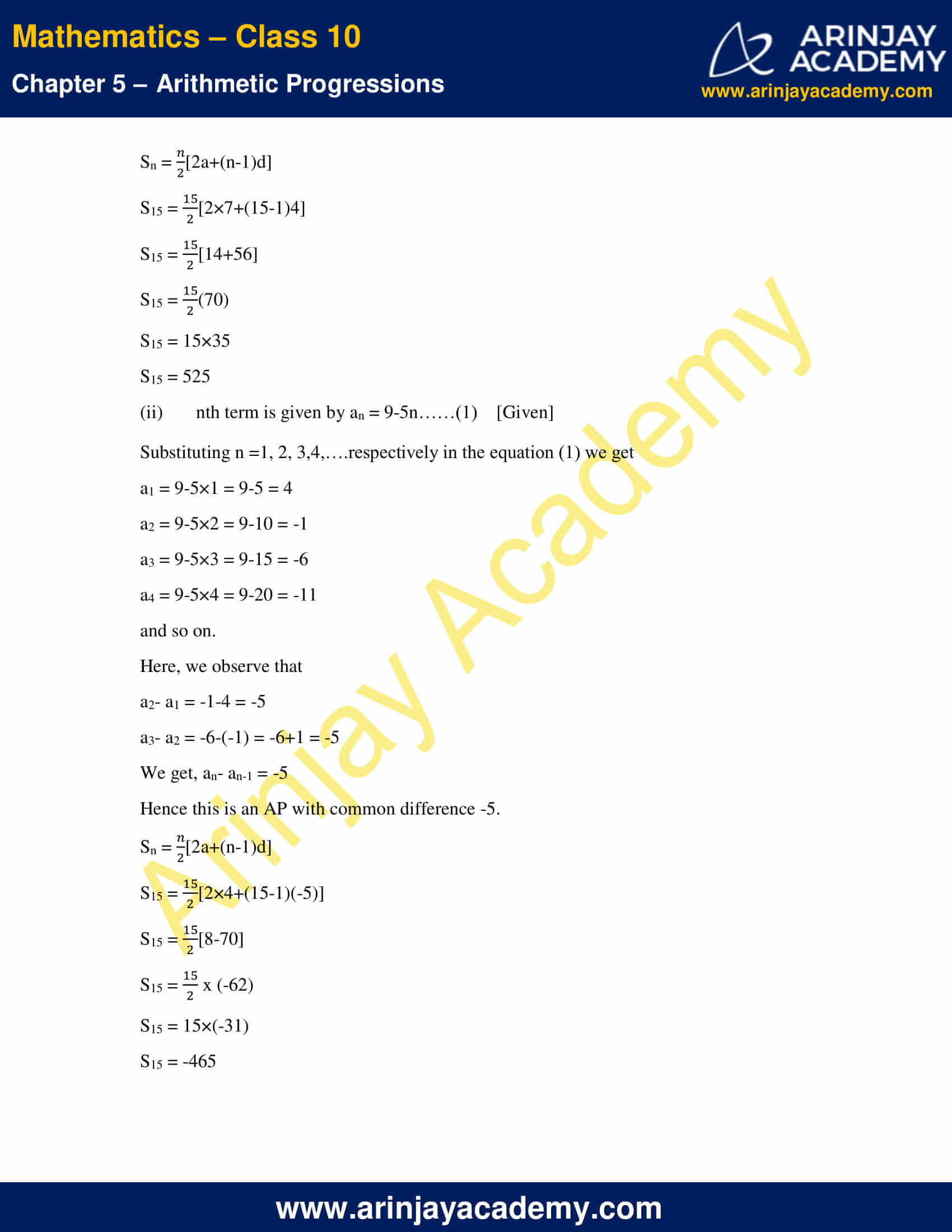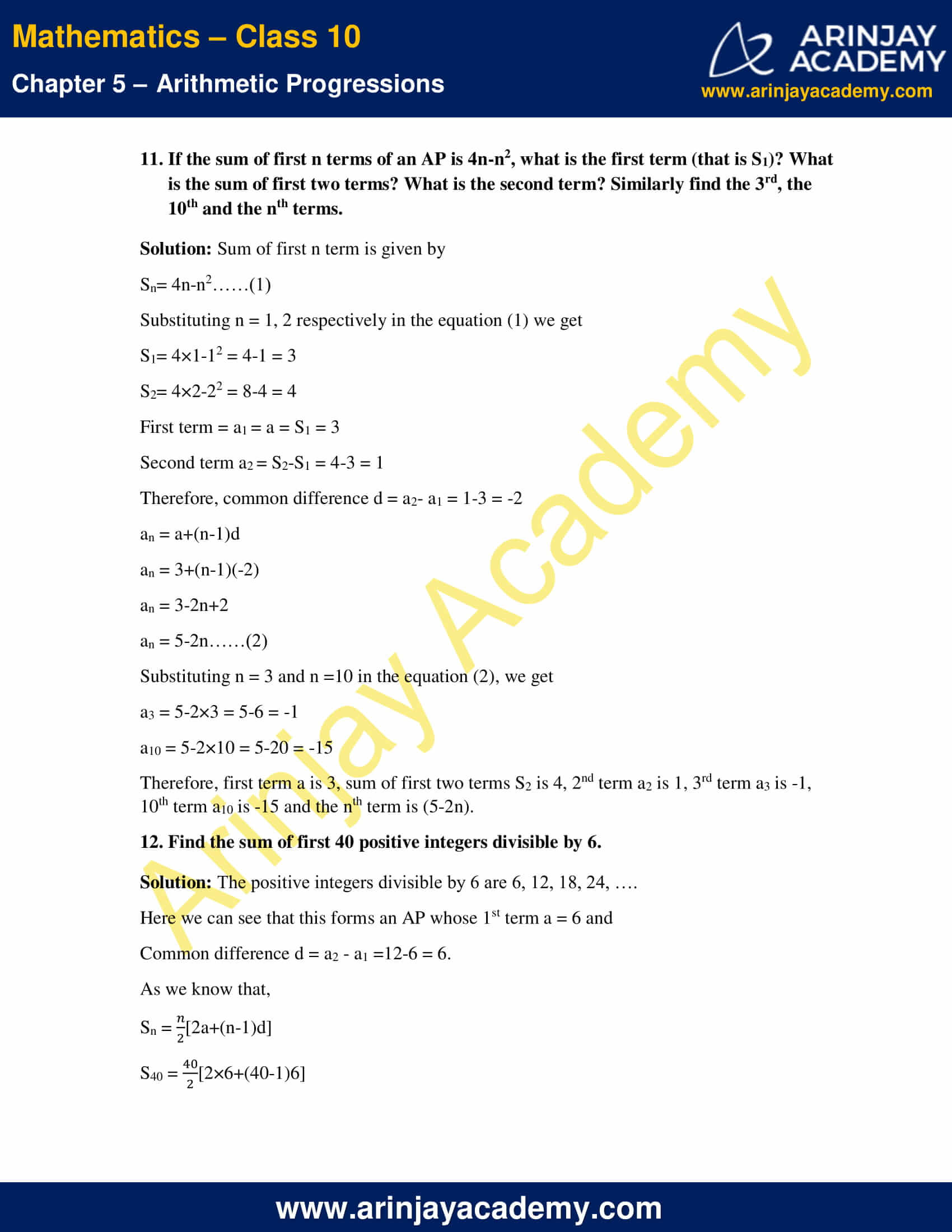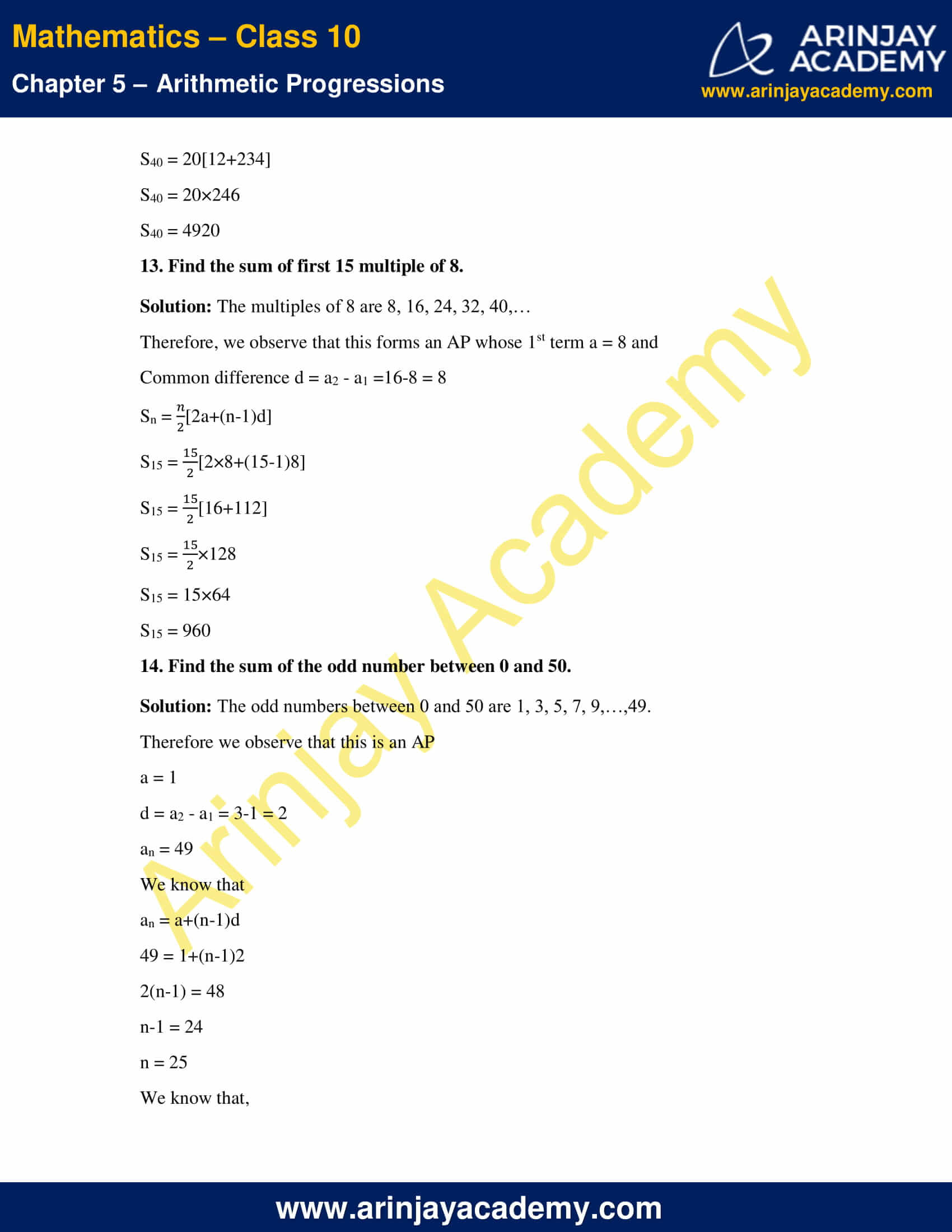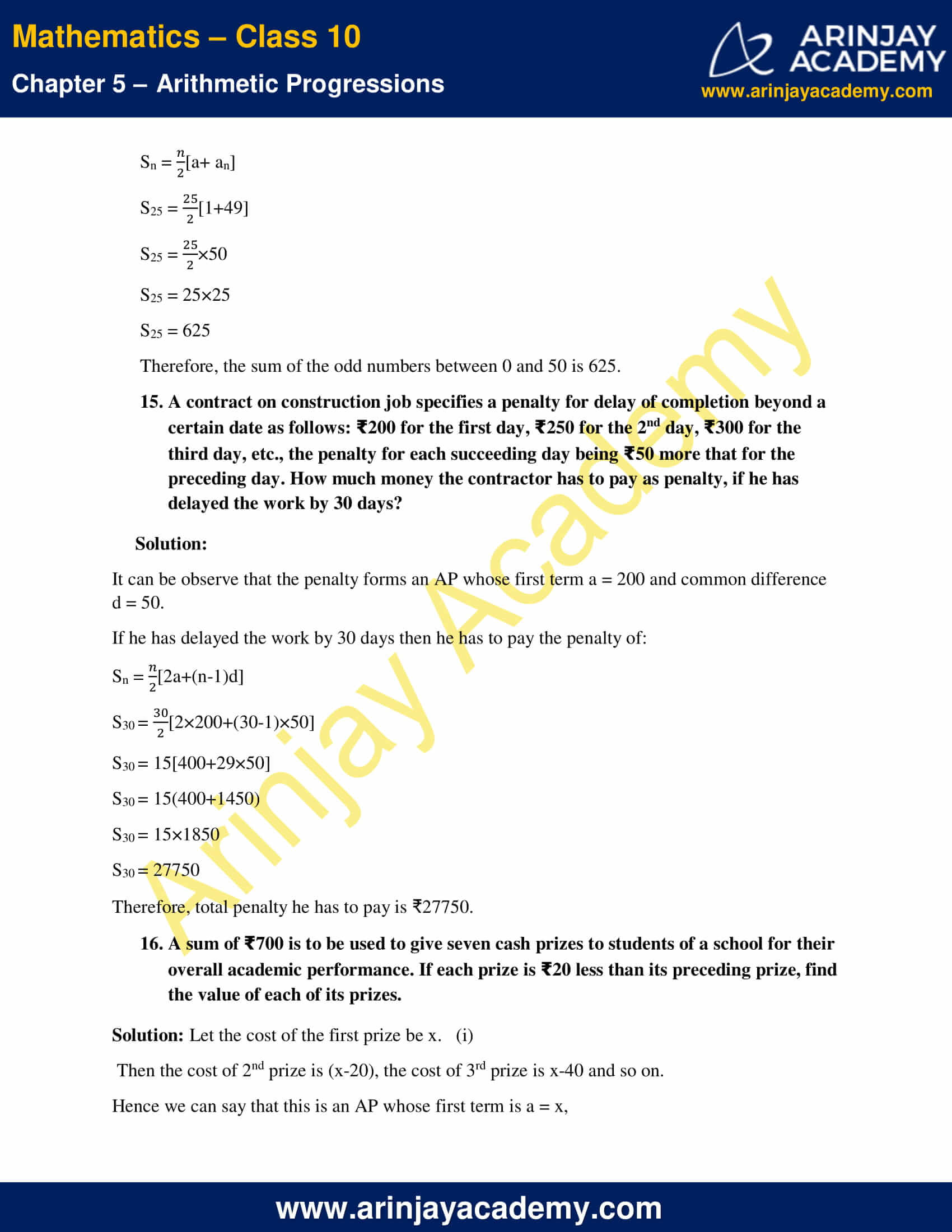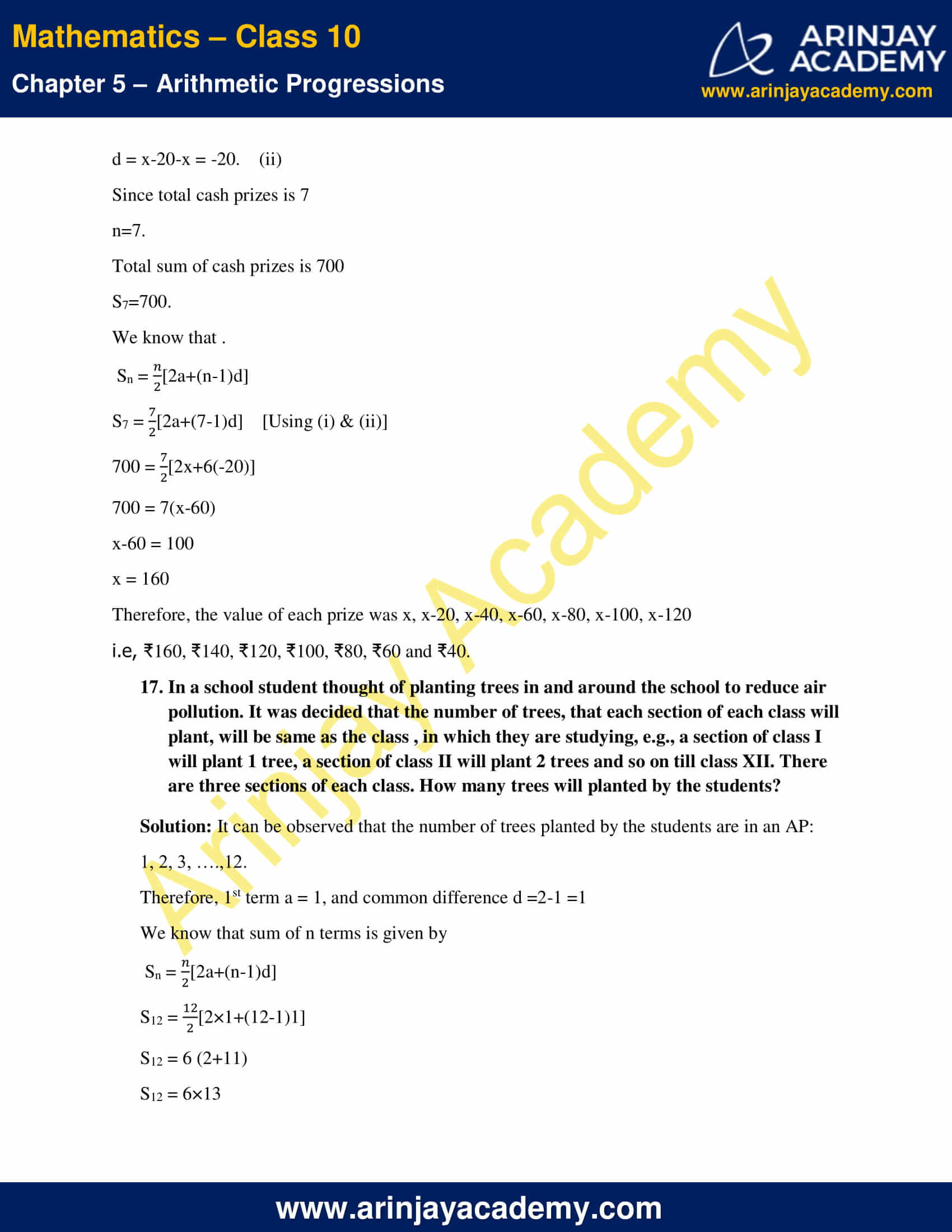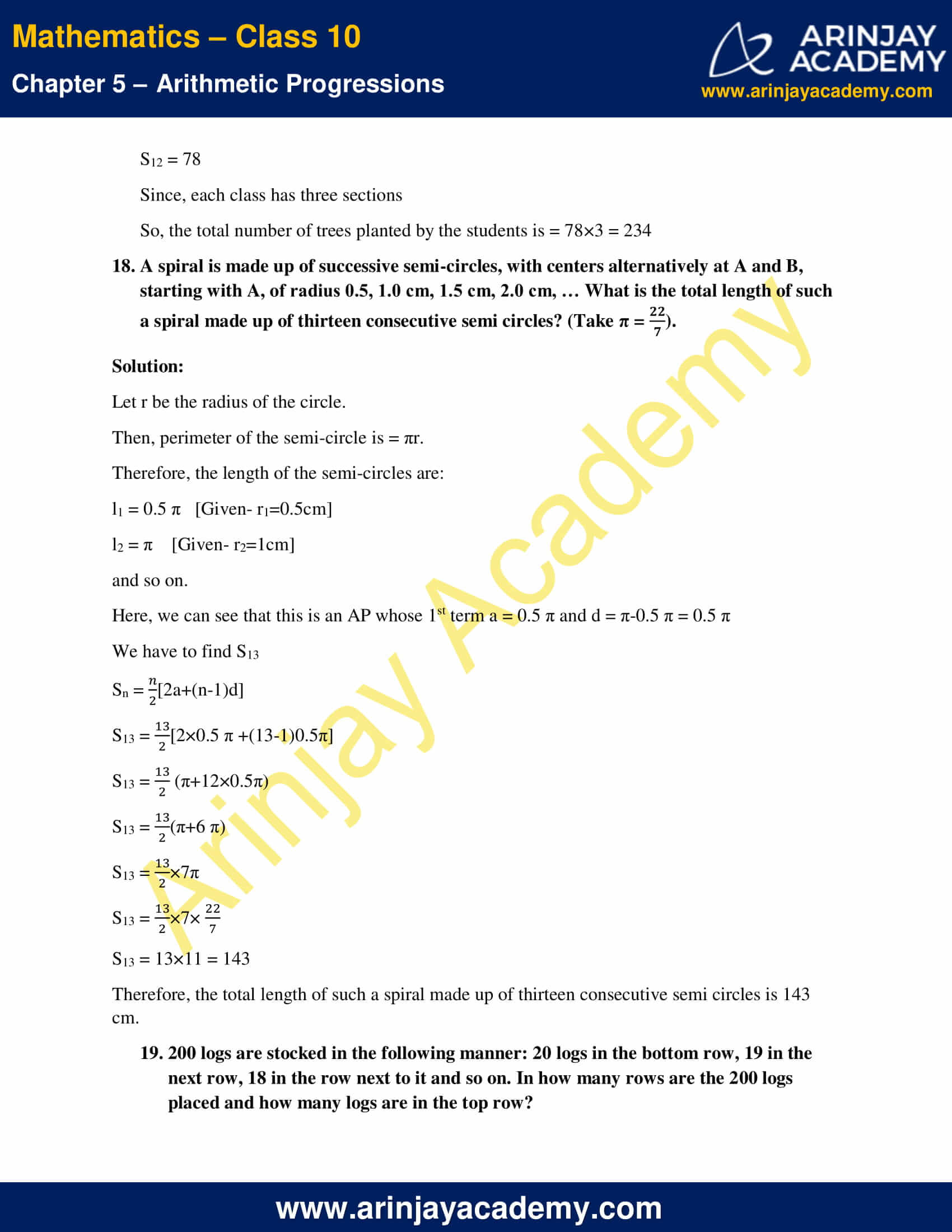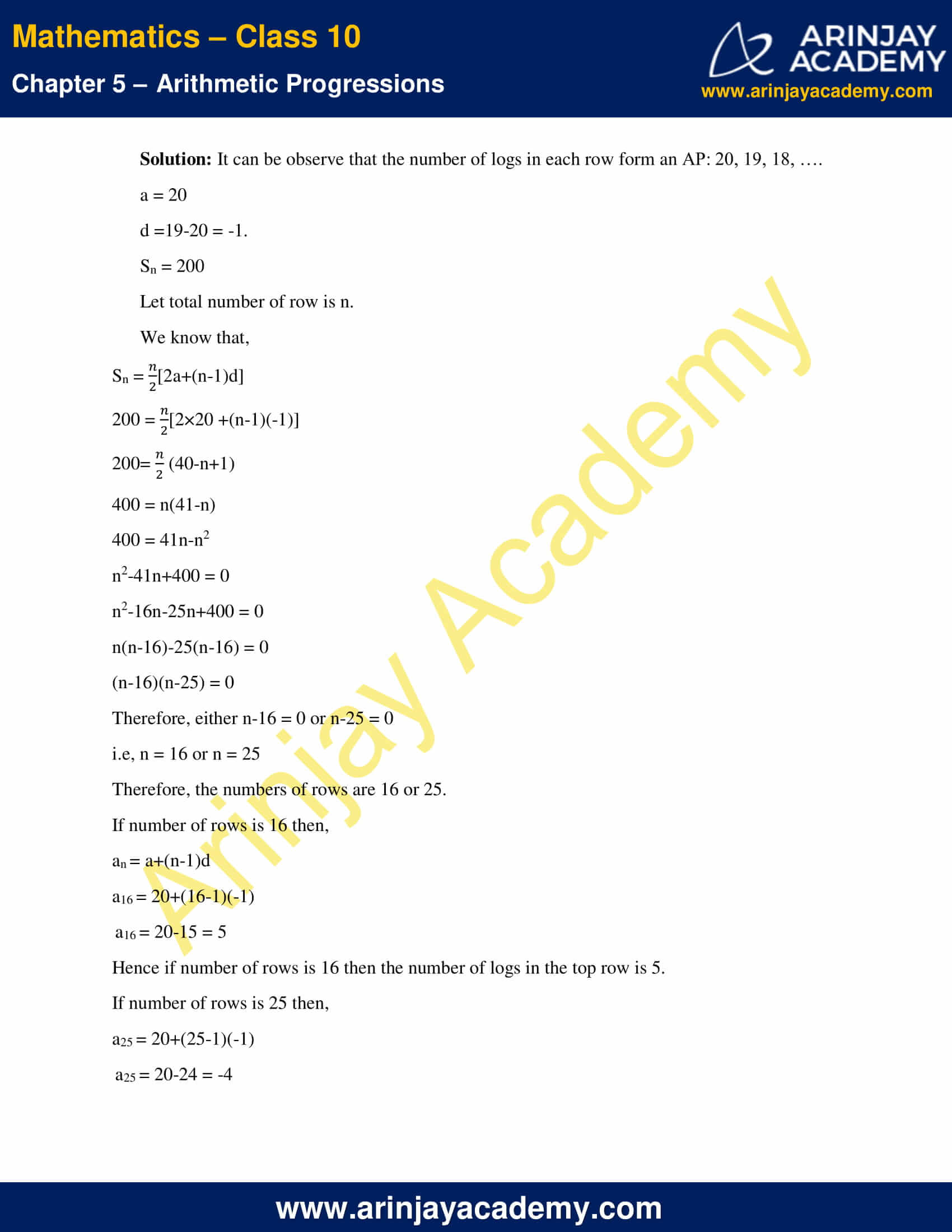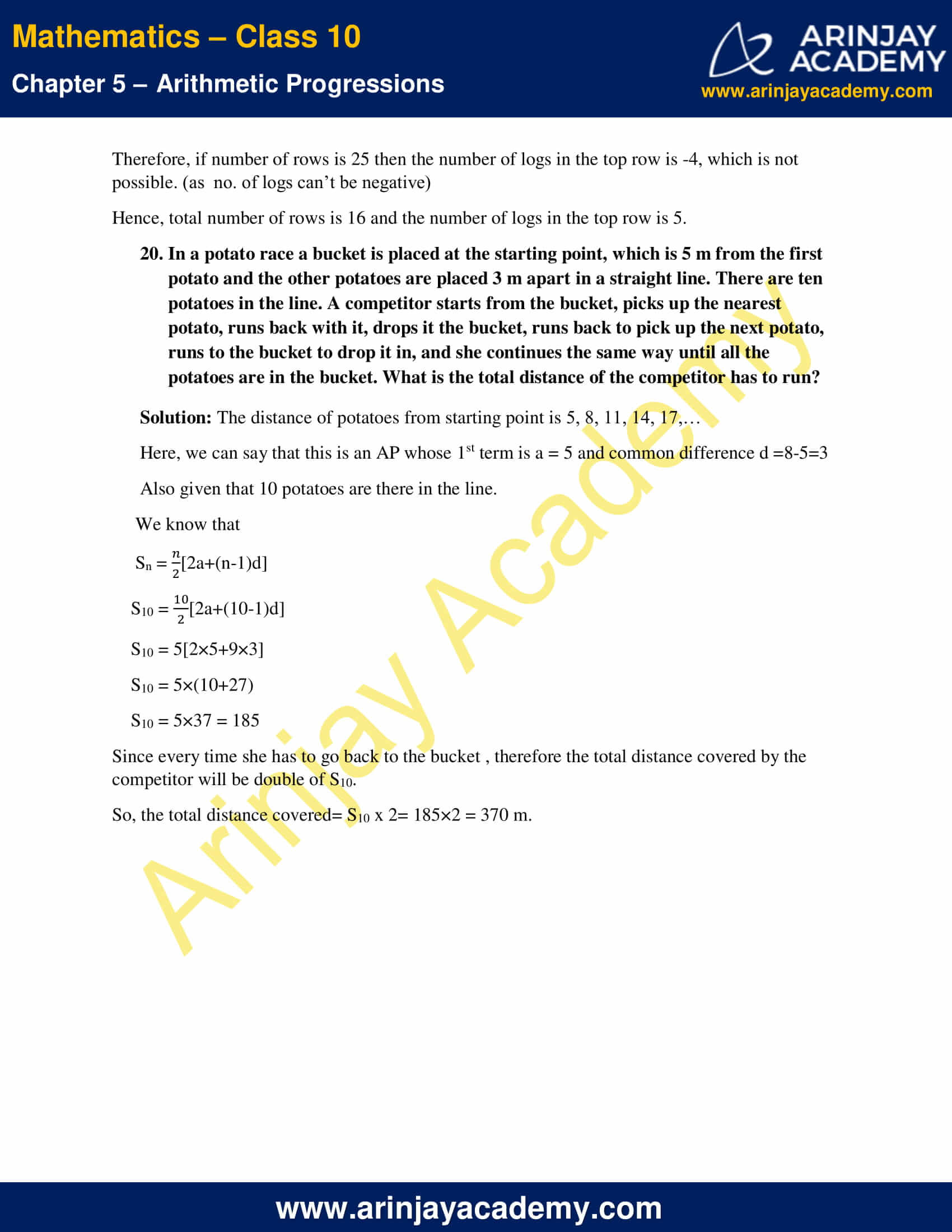NCERT Solutions For Class 10 Maths Chapter 5 Exercise 5.3 – Arithmetic Progressions

1. Find the sum of the following APs.

(i) 2, 7, 12, …. to 10 terms.

Solution:

For this A.P.
a = 2
d = 7-2 = 5
Number of term (n) = 10
As we know that,
Sn = (n/2)[2a+(n-1)d]
S10 = (10/2)[2×2+(10-1)5]
S10 = 5[4+45]
S10 = 245

(ii) -37, -33, -29,…, to 12 terms.

Solution:

For this A.P.
a = -37
d = -33-(-37) = -33+37 = 4
Number of term (n) = 12
As we know that,
Sn = (n/2)[2a+(n-1)d]
S12 = (12/2)[2×(-37)+(12-1)(4)]
S12 = 6[-74+44]
S12 = 6(-30)
S12 = -180

(iii) 0.6, 1.7, 2.8, …, to 100 terms

Solution:
For this A.P.
a = 0.6
d = 1.7-0.6 = 1.1
Number of term n = 100
As we know that,
Sn = (n/2)[2a+(n-1)d]
S100 =(100/2)[2×(0.6)+(100-1)(1.1)]
S100 = 50[1.2+108.9]
S100 = 5505

(iv) (1/15), (1/12) , (1/10) ,…, to 11 terms.

Solution:
For this A.P.
a = (1/15)
d = (1/12) – (1/15) = (5-4)/60 = 1/60
Number of term (n) =11
As we know that,
Sn = (n/2)[2a+(n-1)d]
S11 = (11/2)[2×(1/15)  +(11-1)(1/60)]
S11 = (11/2) [(2/15) +10 x (1/60)]
S11 = (11/2)[(2/15) + (1/6)]
S11= (11/2)×(3/10)
S11 = (33/20)

2. Find the sum of the given below:

(i) 7+10 1⁄2 +14+…+84

Solution:
For this A.P.
a= 7
an= 84
d = 10 1⁄2 -7
d = (21/2) – 7
d = 3 1⁄2
d = 7/2
We know that,
an = a+(n-1)d
7+(n-1)(7/2) = 84
(n-1)(7/2) = 77
n-1 = (77 x 2)/7
n-1 = 22
n = 23
We know that sum of n terms of an AP is
Sn = (n/2)(a +an)
S23 = (23/2)(7+84) = (23/2) x 91 = 1046.5 = 1046(1/2)

(ii) 34+32+30+…+10

Solution:
For this A.P.
a=34
an = 10
d = 32-34 = -2.
We know that,
an = a+(n-1)d
34+(n-1)(-2) = 10
2(n-1) = 24
n-1 = 12
n = 13
We know that sum of n terms of an AP is
Sn = (n/2)(a +an)
S13 = (13/2)(34+10)
= (13/2) x 44
= 286

(iii) -5+(-8)+(-11)+…+(-230)

Solution:
For this A.P.
a= -5
an = -230
d = -8-(-5) = -3
We know that,
an = a+(n-1)d
-5+(n-1)(-3) = -230
3(n-1) = 225
n-1 = 75
n = 76
We know that sum of n terms of an AP is given by
Sn = (n/2)(a +an)
S76 = (76/2)(-5-230)
= (76/2)(-235)
= -8930

3. In an AP

(i) Given a = 5, d = 3, an = 50, find n and Sn

Solution:

We know that,
an = a+(n-1)d
50 = 5+(n-1)3
45 = (n-1)3
15 = n-1
n =16
We know that
Sn = (n/2)(a +an)
Sn = (16/2)(5+50)
Sn = 8×55
Sn = 440
Hence, n = 16 and Sn = 440

(ii) Given a = 7, a13 = 35, find d and S13

Solution:

We know that,
an = a+(n-1)d
35 = 7+12d
28 = 12d
d = (7/3)
We know that,
Sn = (n/2)(a +an)
S13 = (13/2)(7+35)
S13 = (13/2) x 42
S13 = 273.

(iii) Given a12 = 37, d = 3, find a and S12

Solution:

We know that,
an = a+(n-1)d
37 = a + 11×3
37 = a+33
a = 4
We know that,
Sn = (n/2)(a +an)
S12 = (12/2)(4+37)
S12 = 6 x 41
S12 = 246.

(iv) Given a3 = 15, S10 = 125, find d and a10.

Solution:

We know that,
an = a+(n-1)d
15 = a+(3-1)d
15 = a+2d
a+2d = 15……(1)
We know that,
Sn = (n/2)[2a+(n-1)d]
125 = (10/2)(2a+9d)
125 = 5(2a+9d)
2a+9d = 25……(2)
Multiplying equation (1) by 2 we get
2a+4d = 30…..(3)
Subtracting equation (2) from the equation (3) we get
-5d = 5
d = -1
Substituting the value of d in the equation (1) ,we get
a+2(-1) = 15
a = 17
a10 = a+(n-1)d
a10 = 17+9(-1)
a10 = 8.

(v) Given d = 5, S9 = 75, find a and a9

Solution:

We know that
Sn = (n/2)[2a +(n-1)d]
75 = (9/2)[2a+8×5]
2a+40 = (75 x 2)/9
2a+40 = (50/3)
2a = (50/3) – 40
2a = (-70/3)
a = (-35/3)
As we know that,
an = a+(n-1)d
a9 = (-35/3) +8×5
a9 = (-35/3) +40
a9 = (85/3)

(vi) Given a = 2, d= 8, Sn = 90, find n and an

Solution:

We know that,
Sn = (n/2)[2a +(n-1)d]
90 = (n/2)[2×2+(n-1)8]
90 =  (n/2)[4+(n-1)8]
n(8n-4) = 180
8n2-4n-180 = 0
2n2-n-45 = 0
2n2-10n+9n-45 = 0
2n(n-5)+9(n-5) = 0
(n-5)(2n+9) = 0
2n+9 = 0
n = (-9/2) or,
n-5 = 0
n = 5
Since, n can’t be negative or fractional value.
Therefore n = 5
an = a+(n-1)d
a5 = 2+4×8 = 34

(vii) Given a = 8, an = 62, Sn =210, find n and d

Solution:

We know that,
an = a+(n-1)d
62 = 8+(n-1)d
(n-1)d = 54….(1)
We know that,
Sn = (n/2)[a +l]
210 = (n/2)[8+62]
420 = n
n = (420/70)
n = 6
Substituting the value of n in the equation (1), we get
(6-1)d = 54
5d = 54
d = (54/5)

(viii) Given an = 4, d =2, Sn= -14, find n and a.

Solution:

We know that,
an = a+(n-1)d
4 = a+(n-1)2
a+2n = 6
a = 6-2n……(1)
We know that,
Sn = (n/2)[2a +(n-1)d]
-14 = (n/2)[2a+(n-1)2]
-28 = n[2a+2n-2]
On simplifying,
-14 = n(a+n-1)
-14 = n(6-2n+n-1)   (using  (1))
-14 = n(5-n)
n(n-5) = 14
n2-5n-14 = 0
n2-7n+2n-14 = 0
n(n-7)+2(n-7) = 0
(n-7)(n+2) = 0
n+2 = 0
n = -2 or
n-7 = 0
n= 7
Since, n can’t be negative, therefore n = 7
Substituting the value on n in the equation (1), we get
a = 6-2×7
a = 6-14
a = -8

(ix) Given a =3, n =8, S = 192, find d

Solution:

We know that,
Sn = (n/2)[2a +(n-1)d]
192 = (8/2)[2×3+7d]
192 =  4(6+7d)
48 = 6+7d
7d = 42
d = 6

(x) Given l =28, S = 144, and there are total 9 terms. Fine a.

Solution:

Sn = (n/2)[a+l]
144 = (9/2)(a+28)
32 = a+28
a = 4

4. How many terms of the AP: 9, 17, 25,…must be taken to give a sum 636?

Solution:

In the given AP,
a = 9
d = 17-9 = 8
Let the required term be n.
We know that
Sn = (n/2)[2a +(n-1)d]
636 = (n/2)[2×9+(n-1)8]
636 =  (n/2)(18+8n-8)
636 = n(4n+5)
4n2+5n-636 = 0
4n2+53n-48n-636 = 0
n(4n+53)-12(4n+53) = 0
(4n+53)(n-12) = 0
n-12 = 0
n =12 or
4n+53 = 0
n = (-53/4)
Since n can’t be negative or fractional number, therefore n =12
Hence, required term is 12.

5. The first term of an AP is 5, the last term is 45 and the sum is 400. Find the number of terms and the common difference.

Solution:

Let n be the number of term.
Sn = (n/2)[a+l],
400 = (n/2)[5+45]
400 = (n/2) x 50
n = (400 x 2)/50
n = 16      (i)
Now if d be the common difference then the last term of the AP is given by
a+(n-1)d = l
5+15d = 45   Using (i)
15d = 40
d = (8/3)
Hence, the number of term n is 16 and the common difference d is  (8/3).

6. The first and last terms of an AP are 17 and 350 respectively. If the common difference is 9, how many terms are there and what is their sum?

Solution:

Let n be the number of terms in given A.P.
We know that,
an= a+(n-1)d
350 = 17+(n-1)9
333 = (n-1)9
n-1 = 37
n = 38
We know that,
Sn = (n/2)[a+l]
S38= (38/2)(17+350)
S38=6973

7. Find the sum of first 22 terms of an AP in which d =7 and 22nd term is 149.

Solution:

a22=149
Common difference (d) =7
Let first term be a.
We know that,
an= a+(n-1)d
a22= a+(22-1)7
149 = a+21×7
149 = a+147
a = 2
Sum of n terms is given by:
Sn = (n/2)[a+l]
S22= (22/2)[2+149]
S22 = 11×151
S22 = 1661.

8. Find the sum of 1st 51 term of an AP whose 2nd and 3rd terms are 14 and 18 respectively.

Solution:

Given: a2 = 14 and a3= 18
d = a3 –a2 = 18-14 = 4
We know that
an=a+(n-1)d
a2=a+(2-1)d
14 = a+4
a = 10
Sum of n terms of the series is given by:
Sn = (n/2)[2a+(n-1)d]
S51 = (51/2)[2×10+(51-1)4]
S51 = (51/2)[20+200]
S51 = (51/2)×220
S51 = 51×110
S51 = 5610

9. If the sum of first 7 terms of an AP is 49 and that of 17 terms is 289, find the sum of first n terms.

Solution:

We know that,
Sn = (n/2)[2a+(n-1)d]
S7 = (7/2)[2a+(7-1)d]
49 = (7/2)[2a+6d]
49 = 7(a+3d)
a+3d = 7…..(1)
S17 = (17/2)[2a+(17-1)d]
289 = (17/2)[2a+16d]
289 = 17(a+8d)
a+8d = 17…..(2)
Subtracting equation (2) from equation (1) we get
-5d=-10
5d = 10
d = 2
Substituting the value of d in the equation (1) we get
a+3×2 = 7
a+6 = 7
a = 1
Therefore the sum of first n terms of this AP is
Sn = (n/2)[2a+(n-1)d]
Sn = (n/2)[2×1+(n-1)2]
Sn = (n/2)[2+2n-2]
Sn = (n/2) x 2n
Sn = n2

10. Show that a1, a2, ….an,…form an AP where an is defined as below:

(i) an = 3+4n
(ii) an = 9-5n

Also find the sum of the first 15 terms in each case.

Solution:

(i) nth term is given by an = 3+4n……(1) [Given]
Substituting n =1, 2, 3,….respectively in the equation (1) we get
a1 = 3+4×1 = 3+4 = 7
a2 = 3+4×2 = 3+8 = 11
a3 = 3+4×3 = 3+12 = 15
and so on.
Here, we observe that
a2– a1 = 11-7 = 4
a3– a2 = 15-11 = 4
we get, an– an-1 = 4
Hence this is an AP with common difference 4.
Sn = (n/2)[2a+(n-1)d]
S15 = (15/2)[2×7+(15-1)4]
S15 = (15/2)[14+56]
S15 = (15/2)(70)
S15 = 15×35
S15 = 525

(ii) nth term is given by an = 9-5n……(1) [Given]
Substituting n =1, 2, 3,4,….respectively in the equation (1) we get
a1 = 9-5×1 = 9-5 = 4
a2 = 9-5×2 = 9-10 = -1
a3 = 9-5×3 = 9-15 = -6
a4 = 9-5×4 = 9-20 = -11
and so on.
Here, we observe that
a2– a1 = -1-4 = -5
a3– a2 = -6-(-1) = -6+1 = -5
We get, an– an-1 = -5
Hence this is an AP with common difference -5.
Sn = (n/2)[2a+(n-1)d]
S15 = (15/2)[2×4+(15-1)(-5)]
S15 = (15/2)[8-70]
S15 = (15/2) x (-62)
S15 = 15×(-31)
S15 = -465

11. If the sum of first n terms of an AP is 4n-n2, what is the first term (that is S1)? What is the sum of first two terms? What is the second term? Similarly find the 3rd, the 10th and the nth

Solution: Sum of first n term is given by
Sn= 4n-n2……(1)
Substituting n = 1, 2 respectively in the equation (1) we get
S1= 4×1-12 = 4-1 = 3
S2= 4×2-22 = 8-4 = 4
First term = a1 = a = S1 = 3
Second term a2 = S2-S1 = 4-3 = 1
Therefore, common difference d = a2– a1 = 1-3 = -2
an = a+(n-1)d
an = 3+(n-1)(-2)
an = 3-2n+2
an = 5-2n……(2)
Substituting n = 3 and n =10 in the equation (2), we get
a3 = 5-2×3 = 5-6 = -1
a10 = 5-2×10 = 5-20 = -15
Therefore, first term a is 3, sum of first two terms S2 is 4, 2nd term a2 is 1, 3rd term a3 is -1, 10th term a10 is -15 and the nth term is (5-2n).

12. Find the sum of first 40 positive integers divisible by 6.

Solution: The positive integers divisible by 6 are 6, 12, 18, 24, ….
Here we can see that this forms an AP whose 1st term a = 6 and
Common difference d = a2 – a1 =12-6 = 6.
As we know that,
Sn = (n/2)[2a+(n-1)d]
S40 = (40/2)[2×6+(40-1)6]
S40 = 20[12+234]
S40 = 20×246
S40 = 4920

13. Find the sum of first 15 multiple of 8.

Solution: The multiples of 8 are 8, 16, 24, 32, 40,…
Therefore, we observe that this forms an AP whose 1st term a = 8 and
Common difference d = a2 – a1 =16-8 = 8
Sn = (n/2)[2a+(n-1)d]
S15 = (15/2)[2×8+(15-1)8]
S15 = (15/2)[16+112]
S15 = (15/2)×128
S15 = 15×64
S15 = 960

14. Find the sum of the odd number between 0 and 50.

Solution: The odd numbers between 0 and 50 are 1, 3, 5, 7, 9,…,49.
Therefore we observe that this is an AP
a = 1
d = a2 – a1 = 3-1 = 2
an = 49
We know that
an = a+(n-1)d
49 = 1+(n-1)2
2(n-1) = 48
n-1 = 24
n = 25
We know that,
Sn = (n/2)[a+ an]
S25 = (25/2)[1+49]
S25 = (25/2)×50
S25 = 25×25
S25 = 625
Therefore, the sum of the odd numbers between 0 and 50 is 625.

15. A contract on construction job specifies a penalty for delay of completion beyond a certain date as follows: 200 for the first day, 250 for the 2nd day, 300 for the third day, etc., the penalty for each succeeding day being 50 more that for the preceding day. How much money the contractor has to pay as penalty, if he has delayed the work by 30 days?

Solution:

It can be observe that the penalty forms an AP whose first term a = 200 and common difference d = 50.
If he has delayed the work by 30 days then he has to pay the penalty of:
Sn = (n/2)[2a+(n-1)d]
S30 = (30/2)[2×200+(30-1)×50]
S30 = 15[400+29×50]
S30 = 15(400+1450)
S30 = 15×1850
S30 = 27750
Therefore, total penalty he has to pay is ₹27750.

16. A sum of 700 is to be used to give seven cash prizes to students of a school for their overall academic performance. If each prize is 20 less than its preceding prize, find the value of each of its prizes.

Solution: Let the cost of the first prize be x.   (i)
Then the cost of 2nd prize is (x-20), the cost of 3rd prize is x-40 and so on.
Hence we can say that this is an AP whose first term is a = x,
d = x-20-x = -20.    (ii)
Since total cash prizes is 7
∴n=7
Total sum of cash prizes is 700
∴S7=700
We know that .
Sn = (n/2)[2a+(n-1)d]
S7 = (7/2)[2a+(7-1)d]    [Using (i) & (ii)]
700 = (7/2)[2x+6(-20)]
700 = 7(x-60)
x-60 = 100
x = 160
Therefore, the value of each prize was x, x-20, x-40, x-60, x-80, x-100, x-120
i.e, ₹160, ₹140, ₹120, ₹100, ₹80, ₹60 and ₹40.

17. In a school student thought of planting trees in and around the school to reduce air pollution. It was decided that the number of trees, that each section of each class will plant, will be same as the class , in which they are studying, e.g., a section of class I will plant 1 tree, a section of class II will plant 2 trees and so on till class XII. There are three sections of each class. How many trees will planted by the students?

Solution: It can be observed that the number of trees planted by the students are in an AP:
1, 2, 3, ….,12.
Therefore, 1st term a = 1, and common difference d =2-1 =1
We know that sum of n terms is given by
Sn = (n/2)[2a+(n-1)d]
S12 = (12/2)[2×1+(12-1)1]
S12 = 6 (2+11)
S12 = 6×13
S12 = 78
Since, each class has three sections
So, the total number of trees planted by the students is = 78×3 = 234

18. A spiral is made up of successive semi-circles, with centers alternatively at A and B, starting with A, of radius 0.5, 1.0 cm, 1.5 cm, 2.0 cm, … What is the total length of such a spiral made up of thirteen consecutive semi circles? (Take π = (22/7) ).

Solution:

Let r be the radius of the circle.
Then, perimeter of the semi-circle is = πr.
Therefore, the length of the semi-circles are:
l1 = 0.5 π   [Given- r1=0.5cm]
l2 = π    [Given- r2=1cm]
and so on.
Here, we can see that this is an AP whose 1st term a = 0.5 π and d = π-0.5 π = 0.5 π
We have to find S13
Sn = (n/2)[2a+(n-1)d]
S13 = (13/2)[2×0.5 π +(13-1)0.5π]
S13 = (13/2)(π+12×0.5π)
S13 = (13/2)(π+6 π)
S13 = (13/2)×7π
S13 = (13/2)×7×(22/7)
S13 = 13×11 = 143
Therefore the total length of such a spiral made up of thirteen consecutive semi circles is 143 cm.

19. 200 logs are stocked in the following manner: 20 logs in the bottom row, 19 in the next row, 18 in the row next to it and so on. In how many rows are the 200 logs placed and how many logs are in the top row?

Solution: It can be observe that the number of logs in each row form an AP: 20, 19, 18, ….
a = 20
d =19-20 = -1
Sn = 200
Let total number of row is n.
We know that,
Sn = (n/2)[2a+(n-1)d]
200 = (n/2)[2×20 +(n-1)(-1)]
200= (n/2)(40-n+1)
400 = n(41-n)
400 = 41n-n2
n2-41n+400 = 0
n2-16n-25n+400 = 0
n(n-16)-25(n-16) = 0
(n-16)(n-25) = 0
Therefore, either n-16 = 0 or n-25 = 0
i.e, n = 16 or n = 25
Therefore, the numbers of rows are 16 or 25.
If number of rows is 16 then,
an = a+(n-1)d
a16 = 20+(16-1)(-1)
a16 = 20-15 = 5
Hence if number of rows is 16 then the number of logs in the top row is 5.
If number of rows is 25 then,
a25 = 20+(25-1)(-1)
a25 = 20-24 = -4
Therefore, if number of rows is 25 then the number of logs in the top row is -4, which is not possible. (as  no. of logs can’t be negative)
Hence, total number of rows is 16 and the number of logs in the top row is 5.

20. In a potato race a bucket is placed at the starting point, which is 5 m from the first potato and the other potatoes are placed 3 m apart in a straight line. There are ten potatoes in the line. A competitor starts from the bucket, picks up the nearest potato, runs back with it, drops it the bucket, runs back to pick up the next potato, runs to the bucket to drop it in, and she continues the same way until all the potatoes are in the bucket. What is the total distance of the competitor has to run?

Solution: The distance of potatoes from starting point is 5, 8, 11, 14, 17,…
Here, we can say that this is an AP whose 1st term is a = 5 and common difference d =8-5=3
Also given that 10 potatoes are there in the line.
We know that
Sn = (n/2)[2a+(n-1)d]
S10 = (10/2)[2a+(10-1)d]
S10 = 5[2×5+9×3]
S10 = 5×(10+27)
S10 = 5×37 = 185
Since every time she has to go back to the bucket , therefore the total distance covered by the competitor will be double of S10.
So, the total distance covered= S10 x 2= 185×2 = 370 m.

NCERT Solutions for Class 10 Maths Chapter 5 Exercise 5.3 – Arithmetic Progressions, has been designed by the NCERT to test the knowledge of the student on the topic – Sum of First n Terms of an AP

NCERT Solutions For Class 10 Maths Chapter 5 Exercise 5.3 – Arithmetic Progressions